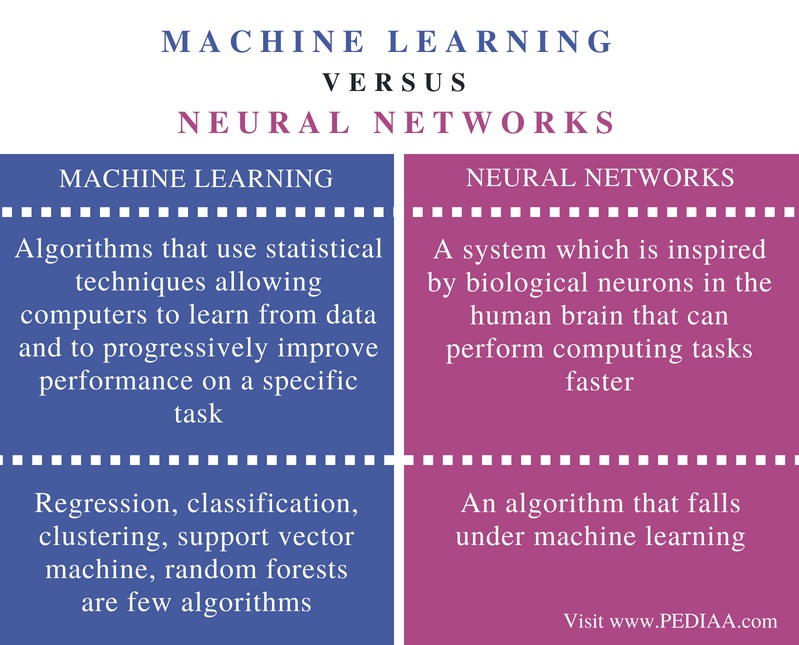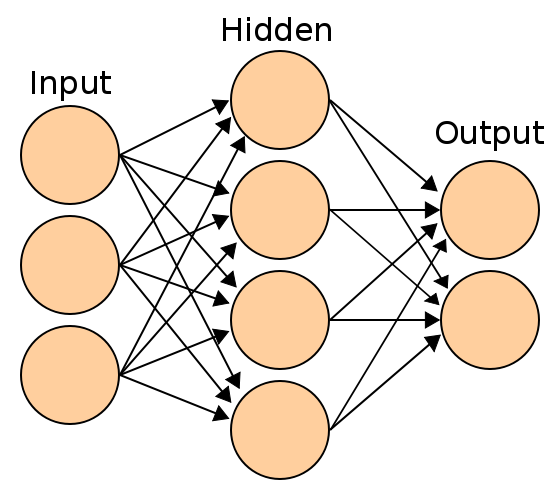# Difference Between Machine Learning and Neural Networks

The main difference between machine learning and neural networks is that the machine learning refers to developing algorithms that can analyze and learn from data to make decisions while the neural networks is a group of algorithms in machine learning that perform computations similar to neurons in the human brain.

Machine learning is the technique of developing self-learning algorithms that can analyze data, learn from them, recognize patterns and make decisions accordingly. It is a subcategory of Artificial Intelligence. Machine learning uses various algorithms. Neural network is one of them. These concepts are broadly used in various fields such as medicine, robotics, manufacturing, and agriculture.

### Key Areas Covered

1. What is Machine Learning
– Definition, Types, Functionality
2. What is Neural Networks
– Definition, Types, Functionality
3. Difference Between Machine Learning and Neural Networks
– Comparison of Key Differences

### Key Terms

Artificial Intelligence, Feedback Network, Feedforward Network, Machine Learning, Neural Networks, Supervised Learning, Unsupervised Learning## What is Machine Learning

Machine learning is a subset of Artificial Intelligence. Machine learning algorithms analyze data, learn from them and make decisions. It uses statistical methods and allows the machine to improve with experience.Figure 1: Machine Learning

There are two main types of machine learning: supervised learning and unsupervised learning. In supervised learning, there are input variables (x) and output variables (y). The algorithm is trained by mapping the inputs to the outputs (y=f(x)). When providing a new input, the algorithm should predict the output. Linear regression, support vector machine and random forests are some examples of supervised learning.

In unsupervised learning, there is only input data (x). There is no output data. In this type, it is not necessary to train the algorithm. Instead, it discovers the patterns in the input data on its own. One main unsupervised learning algorithm is clustering. It identifies the similar instances and groups them together to create clusters. Usually, unsupervised learning is difficult than supervised learning. In brief, machine learning helps to develop systems that can learn and perform predictions using data.

## What are Neural Networks

Neural networks are inspired by biological neurons. In the human brain, there are millions of neurons and the information passes from one neuron to the other. Neural networks use this concept to perform computational tasks faster.Figure 2: Neural Network

There are two types of neural networks called feedforward and feedback. In feedforward networks, the information passes only from the input to the output and it does not contain a feedback loop. In feedback networks, the information can pass to both directions and it contains a feedback path.

The feedforward networks further are categorized into single layer network and multi-layer network. In single layer network, the input layer connects to the output layer. On the other hand, the multi-layer network has more layers called hidden layers between the input layer and output layer.

A neural network contains nodes. These nodes are similar to the neurons in the brain. Furthermore, the connections in the network have specific weights. When the inputs to the nodes are x1, x2, x3… and corresponding weights are w1, w2, w3, … the net input (y) is similar to the following.

y= x1. w1 + x2. w2+x3.w3+….

After applying the activation function such as linear or a sigmoid to the net input, it provides the output as below.

Y= F(y)

Then, the output is evaluated. The weights adjust if the evaluated output is different from the desired output. This process is repeated until the desired outputs are obtained. This is the basic functionality of a neural network.

## Difference Between Machine Learning and Neural Networks

### Definition

Machining learning refers to algorithms that use statistical techniques allowing computers to learn from data and to progressively improve performance on a specific task. A neural network is a system which is inspired by biological neurons in the human brain that can perform computing tasks faster.

### Algorithms

Regression, classification, clustering, support vector machine, random forests are few algorithms in machine learning. Neural networks are also an algorithm that falls under machine learning.

### Conclusion

The difference between machine learning and neural networks is that the machine learning refers to developing algorithms that can analyze and learn from data to make decisions while the neural networks is a group of algorithms in machine learning that perform computations similar to neutrons in the human brain.

##### Reference:

1. What Is Machine Learning? | Machine Learning Basics | Machine Learning Tutorial | Edureka!, 16 Mar. 2018, Available here.

##### Image Courtesy:

1. “3161590” (CC0) via Pixabay
2. “Artificial neural network” By en:User:Cburnett – Own workThis vector image was created with Inkscape (CC BY-SA 3.0) via Commons Wikimedia### About the Author: Lithmee

Lithmee holds a Bachelor of Science degree in Computer Systems Engineering and is reading for her Master’s degree in Computer Science. She is passionate about sharing her knowldge in the areas of programming, data science, and computer systems.